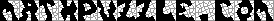This header plots the critical line of the Riemann Zeta Function.  A complete understanding wins a \$1,000,000 prize.
 . . .Main Links Orders Post Next Page Next + 10

UNSOLVED DIOPHANTINE EQUATIONS

1.  For a million dollars, solve the Birch and Swinnerton-Dyer Conjecture.  Description by Andrew Wiles.  (requires Acrobat Reader).

2.  The Beal Conjecture.  If Ax +By = Cz , where A, B, C, x, y and z are positive integers and x, y and z are all greater than 2, then A, B and C must have a common factor.  \$75,000 prize.

3.  Is there a 3x3 magic square, with 9 distinct entries, so that every entry is a square?   Martin Gardner offers \$100 for a resolution of this question.

4.  Catalan Problem:  a^b - c^d = 1    Is there a solution besides a = d = 3, b = c = 2 (3^2 - 2^3 = 1)?

5.  1^n + 2^n +... + k^n = (k+1)^n    Solution for n>1 ?

6.  (x+y+z)^3 = xyz   Non-trivial solutions?  (Sierpinski)

7.  Euler's Brick.  Is there a Integral Rect. Parallelepiped?  a^2 + b^2 = c^2    a^2 + d^2 = e^2    b^2 + d^2 = f^2   b^2 + e^2 = g^2

8.  Characterize those d for which x^2 - dy^2 = -1 has a solution.

Mathworld has an extensive page on Diophantine Equations.
http://www.math.niu.edu/~rusin/papers/known-math/index/11DXX.html
http://sisenis.com.latnet.lv/~podnieks/gt4.html
http://www.mathsoft.com/asolve/fermat/fermat.html